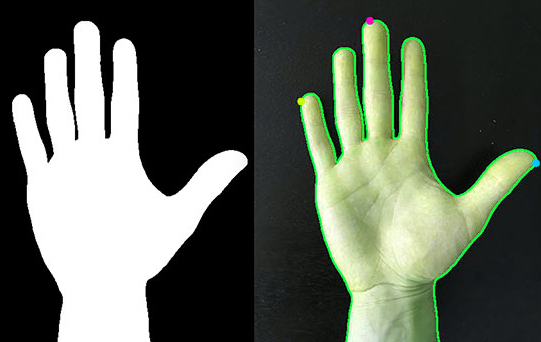• +91-9872993883
• +91-8283824812
• info@ris-ai.com

# Hand Gesture Recognition with Python¶

### Overview of hand gesture recognition using python

In the recent few years , Hand Gesture Recognition with Python is a system that can detect the gesture of hand in a real time video.Hand tracking and segmentation are the primary steps for any hand gesture recognition system.

This system has been applied for different applications in different fields including; translation into sign language , virtual environments, intelligent monitoring , robot control , medical systems , etc.

Now let’s see how to train a Machine Learning model in Hand Gesture Recognition with Python programming language. We will start with importing the necessary libraries and reading the datasets that we need for this task:In :

### import python libraries

import numpy as np # linear algebra
import pandas as pd # data processing

df = pd.concat([df0,df1,df2,df3], axis = 0)

In :
x = df.loc[:,0:63]
y = df

In :
x.head()

Out:
0 1 2 3 4 5 6 7 8 9 ... 54 55 56 57 58 59 60 61 62 63
0 26.0 4.0 5.0 8.0 -1.0 -13.0 -109.0 -66.0 -9.0 2.0 ... 21.0 -28.0 61.0 4.0 8.0 5.0 4.0 -7.0 -59.0 16.0
1 -47.0 -6.0 -5.0 -7.0 13.0 -1.0 35.0 -10.0 10.0 -4.0 ... -105.0 -25.0 47.0 6.0 6.0 5.0 13.0 21.0 111.0 15.0
2 -19.0 -8.0 -8.0 -8.0 -21.0 -6.0 -79.0 12.0 0.0 5.0 ... -128.0 -83.0 7.0 7.0 1.0 -8.0 7.0 21.0 114.0 48.0
3 2.0 3.0 0.0 2.0 0.0 22.0 106.0 -14.0 -16.0 -2.0 ... -54.0 -38.0 -11.0 4.0 7.0 11.0 33.0 39.0 119.0 43.0
4 6.0 0.0 0.0 -2.0 -14.0 10.0 -51.0 5.0 7.0 0.0 ... 60.0 38.0 -35.0 -8.0 2.0 6.0 -13.0 -24.0 -112.0 -69.0

5 rows × 64 columns

#### Now we will split the data into 75% training and 25% test set:

In :
from sklearn.model_selection import train_test_split
x_train, x_test, y_train, y_test = train_test_split(x, y, test_size=0.25, random_state=42)


#### Now We will rescale the data using Standard Scalar:

In :
from sklearn.preprocessing import StandardScaler
sc = StandardScaler()
x_train = pd.DataFrame(sc.fit_transform(x_train))
x_test = pd.DataFrame(sc.transform(x_test))


Now We will use the Random Forest Classifier to train a Hand Gesture Recognition model with Python:

In :
from sklearn.ensemble import RandomForestClassifier
from sklearn.model_selection import GridSearchCV

lr_grid = {'max_depth' : [4,8,16,32,64,128],
'criterion' : ['entropy','gini']}

clf = RandomForestClassifier(n_estimators=100, max_features='sqrt', random_state=42)

gs = GridSearchCV(estimator = clf, param_grid=lr_grid,cv = 5)
gs.fit(x_train,y_train)
y_pred = gs.predict(x_test)
gs.best_params_

Out:
{'criterion': 'entropy', 'max_depth': 32}

Now let’s check the accuracy of the model using the confusion matrix and print the classification report of our machine learning model:

In :
from sklearn.metrics import classification_report
print('Classification Report: \n', classification_report(y_test,y_pred))

Classification Report:
precision    recall  f1-score   support

0       0.94      0.97      0.95       719
1       0.96      0.92      0.94       769
2       0.91      0.95      0.93       703
3       0.89      0.86      0.87       729

accuracy                           0.92      2920
macro avg       0.92      0.93      0.92      2920
weighted avg       0.92      0.92      0.92      2920


In :
from sklearn.metrics import confusion_matrix
print('Confusion Matrix: \n', confusion_matrix(y_test,y_pred))

Confusion Matrix:
[[698   0   7  14]
[  0 709  22  38]
[  4   7 665  27]
[ 43  25  33 628]]

In :
from sklearn.metrics import accuracy_score
accuracy_score(y_test,y_pred)

Out:
0.9246575342465754